## Overview: LP Procedure

The LP procedure solves linear programs, integer programs, and mixed-integer programs. It also performs parametric programming, range analysis, and reports on solution sensitivity to changes in the right-hand-side constants and price coefficients.

The LP procedure provides various control options and solution strategies. It also provides the functionality to produce various kinds of intermediate and final solution information. The procedure’s interactive features enable you to take control of the problem solving process. During linear or integer iterations, for example, you can stop the procedure at intermediate stages and examine current results. If necessary, you can change options or strategies and resume the execution of the procedure.

The LP procedure is used to optimize a linear function subject to linear and integer constraints. Specifically, the LP procedure solves the general mixed-integer program of the form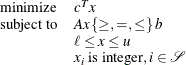where

•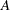is anmatrix of technological coefficients

•is an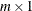matrix of right-hand-side (RHS) constants

•is an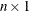matrix of objective function coefficients

•is anmatrix of structural variables

•is anmatrix of lower bounds on•is anmatrix of upper bounds on•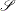is a subset of the set of indices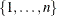Linear programs (whenis empty) are denoted by (LP). For these problems, the procedure employs the two-phase revised simplex method, which uses the Bartels-Golub update of the LU decomposed basis matrix to pivot between feasible solutions (Bartels 1971). In phase 1, PROC LP finds a basic feasible solution to (LP), while in phase 2, PROC LP finds an optimal solution,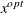. The procedure implicitly handles unrestricted variables, lower-bounded variables, upper-bounded variables, and ranges on constraints. When no explicit lower bounds are specified, PROC LP assumes that all variables are bounded below by zero.

When a variable is specified as an integer variable,has at least one element. The procedure then uses the branch-and-bound technique for optimization.

The relaxed problem (the problem with no integer constraints) is solved initially using the primal algorithm described previously. Constraints are added in defining the subsequent descendant problems in the branch-and-bound tree. These problems are then solved using the dual simplex algorithm. Dual pivots are referred to as phase 3 pivots.

The preprocessing option enables the procedure to identify redundant and infeasible constraints, fix variables, and reduce the feasible region before solving a problem. For linear programs, the option often can reduce the number of constraints and variables, leading to a quicker elapsed solution time and improved reliability. For integer programs, it often reduces the gap between an integer program and its relaxed linear program, which will likely lead to a reduced branch-and-bound tree and a quicker CPU time. In general, it provides users an alternative to solving large, complicated operations research problems.

The LP procedure can also analyze the sensitivity of the solutionto changes in both the objective function and the right-hand-side constants. There are three techniques available for this analysis: sensitivity analysis, parametric programming, and range analysis. Sensitivity analysis enables you to examine the size of a perturbation to the right-hand-side or objective vector by an arbitrary change vector for which the basis of the current optimal solution remains optimal.

Parametric programming, on the other hand, enables you to specify the size of the perturbation beforehand and examine how the optimal solution changes as the desired perturbation is realized. With this technique, the procedure pivots to maintain optimality as the right-hand-side or objective vector is perturbed beyond the range for which the current solution is optimal. Range analysis is used to examine the range of each right-hand-side value or objective coefficient for which the basis of the current optimal solution remains optimal.

The LP procedure can also save both primal and dual solutions, the current tableau, and the branch-and-bound tree in SAS data sets. This enables you to generate solution reports and perform additional analyses with the SAS System. Although PROC LP reports solutions, this feature is particularly useful for reporting solutions in formats tailored to your specific needs. Saving computational results in a data set also enables you to continue executing a problem not solved because of insufficient time or other computational problems.

The LP procedure uses the Output Delivery System (ODS), a SAS subsystem that provides capabilities for displaying and controlling the output from SAS procedures. ODS enables you to modify the headers, column names, data formats, and layouts of the output tables in PROC LP.

There are no restrictions on the problem size in the LP procedure. The number of constraints and variables in a problem that PROC LP can solve depends on the host platform, the available memory, and the available disk space for utility data sets.

You can also solve LP problems by using the OPTLP procedure. The OPTLP procedure requires a linear program to be specified using a SAS data set that adheres to the MPS format, a widely accepted format in the optimization community. You can use the MPSOUT= option in the LP procedure to convert typical PROC LP format data sets into MPS-format SAS data sets.